### Gorillaz - N-art Band TributeHover over the thumbnail for a full-size version.

Author AMomentLikeThis author:amomentlikethis bitesized n-art rated 2005-11-13 4 by 31 people. \$Gorillaz - N-art Band Tribute#AMomentLikeThis#none#00000000000000000000000000000000000000000000000000000000000000000000000000000000000000000000000000000000000000000000000000000000000000000000000000000000000000000000000000000000000000000000000000000000000000000000000000000000000000000000000000000000000000000000000000000000000000000000000000000000000000000000000000000000000000000000000000000000000000000000000000000000000000000000000000000000000000000000000000000000000000000000000000000000000000000000000000000000000000000000000000000000000000000000000000000000000000000000000000000000000000000000000000000000000000000000000000000000000000000000000000000000000000000000000000000000000000000000000000000000000000000000000000000000000000000000000000000000000000000|12^366,222!12^372,216!12^378,222!12^372,222!12^366,228!12^372,228!12^378,228!12^366,234!12^372,234!12^378,234!12^366,252!12^366,246!12^366,240!12^372,240!12^372,246!12^372,252!12^378,240!12^378,246!12^378,252!12^360,258!12^366,258!12^372,258!12^378,258!12^378,264!12^372,264!12^372,270!12^366,276!12^366,270!12^366,264!12^360,264!12^360,270!12^360,270!12^360,276!12^360,282!12^360,288!12^366,282!12^372,276!12^378,270!12^372,282!12^366,288!12^372,288!12^360,294!12^366,294!12^372,294!12^354,300!12^360,300!12^366,300!12^372,300!12^372,306!12^372,312!12^366,312!12^366,306!12^360,306!12^354,306!12^354,312!12^360,312!12^366,318!12^366,324!12^360,318!12^354,318!12^360,324!12^354,324!12^354,330!12^360,330!12^366,330!12^354,336!12^360,336!12^366,336!12^354,342!12^360,342!12^366,342!12^366,348!12^360,348!12^354,348!12^354,354!12^360,354!12^366,354!12^354,360!12^360,360!12^366,360!12^372,360!12^378,360!12^354,366!12^360,366!12^366,366!12^372,366!12^378,366!12^360,372!12^366,372!12^372,372!12^378,372!12^372,354!12^384,372!12^384,366!12^384,360!12^390,360!12^396,360!12^396,366!12^396,372!12^390,372!12^390,366!12^402,372!12^408,378!12^408,372!12^408,366!12^408,360!12^402,360!12^402,366!12^414,360!12^420,360!12^432,360!12^426,360!12^438,366!12^438,372!12^444,372!12^432,378!12^414,378!12^438,378!12^414,372!12^432,372!12^432,372!12^432,366!12^414,366!12^420,372!12^426,372!12^426,378!12^420,378!12^426,366!12^420,366!12^408,336!12^408,330!12^408,324!12^408,318!12^408,312!12^408,306!12^408,300!12^408,294!12^408,288!12^408,282!12^408,276!12^408,270!12^414,264!12^414,258!12^414,252!12^414,246!12^414,240!12^414,234!12^414,228!12^420,222!12^420,216!12^420,210!12^420,204!12^420,198!12^420,192!12^426,186!12^432,192!12^432,198!12^432,204!12^432,210!12^432,216!12^432,222!12^432,228!12^432,234!12^432,240!12^420,228!12^426,228!12^426,222!12^426,216!12^426,210!12^426,204!12^426,198!12^426,192!12^420,240!12^426,234!12^426,240!12^420,234!12^426,246!12^420,246!12^420,252!12^426,252!12^426,258!12^420,258!12^426,264!12^426,270!12^426,276!12^426,282!12^420,282!12^420,276!12^420,270!12^420,264!12^414,270!12^414,276!12^414,282!12^420,288!12^414,288!12^414,300!12^414,294!12^420,294!12^420,300!12^420,306!12^414,306!12^414,312!12^420,312!12^420,318!12^414,318!12^414,324!12^414,330!12^420,324!12^414,336!12^414,342!12^420,336!12^420,330!12^426,324!12^426,330!12^426,336!12^420,342!12^426,342!12^432,342!12^438,342!12^444,342!12^450,342!12^456,342!12^456,348!12^432,336!12^438,336!12^450,336!12^444,336!12^456,336!12^456,330!12^456,324!12^456,318!12^450,324!12^450,330!12^444,324!12^444,330!12^438,324!12^432,324!12^432,330!12^438,330!12^462,312!12^462,306!12^468,300!12^468,294!12^474,288!12^474,282!12^480,276!12^486,270!12^492,264!12^498,264!12^504,258!12^510,258!12^516,252!12^522,252!12^528,258!12^534,258!12^540,264!12^546,264!12^552,270!12^558,276!12^564,276!12^564,270!12^564,264!12^564,252!12^564,258!12^558,246!12^558,240!12^558,228!12^558,234!12^552,222!12^552,216!12^546,210!12^534,198!12^540,204!12^528,198!12^522,192!12^516,192!12^510,186!12^504,186!12^498,192!12^492,192!12^486,198!12^480,198!12^474,204!12^468,210!12^462,216!12^462,222!12^456,228!12^456,234!12^450,240!12^450,246!12^456,252!12^468,246!12^462,246!12^456,246!12^456,240!12^462,240!12^474,240!12^474,234!12^480,228!12^480,222!12^486,216!12^492,210!12^498,204!12^504,204!12^510,204!12^516,204!12^522,210!12^528,210!12^534,216!12^540,222!12^540,228!12^546,234!12^546,240!12^546,246!12^552,252!12^552,258!12^552,264!12^558,270!12^558,264!12^558,258!12^558,252!12^552,246!12^552,240!12^552,234!12^552,228!12^546,228!12^546,222!12^546,216!12^540,216!12^540,210!12^534,210!12^534,204!12^528,204!12^522,204!12^522,198!12^516,198!12^510,198!12^510,192!12^504,192!12^504,198!12^498,198!12^492,198!12^492,204!12^480,204!12^486,204!12^486,210!12^480,210!12^480,216!12^474,216!12^474,210!12^468,216!12^468,222!12^474,222!12^468,228!12^468,234!12^468,240!12^462,234!12^462,228!12^474,228!12^462,354!12^468,360!12^474,366!12^480,372!12^486,372!12^498,378!12^492,378!12^504,384!12^510,384!12^516,378!12^522,378!12^528,372!12^534,372!12^540,366!12^546,360!12^552,354!12^558,348!12^564,348!12^570,342!12^570,336!12^570,330!12^570,318!12^570,324!12^570,312!12^570,306!12^570,300!12^570,294!12^570,288!12^570,282!12^558,282!12^564,288!12^558,288!12^564,282!12^564,294!12^564,306!12^564,312!12^564,324!12^564,336!12^558,342!12^564,342!12^564,330!12^564,318!12^564,300!12^558,294!12^558,300!12^558,312!12^558,306!12^558,324!12^558,330!12^558,336!12^558,318!12^480,318!12^480,312!12^486,306!12^486,300!12^492,294!12^492,288!12^498,282!12^504,276!12^510,276!12^516,270!12^522,270!12^528,276!12^534,276!12^540,282!12^540,288!12^540,294!12^540,300!12^540,306!12^540,312!12^540,318!12^540,324!12^540,330!12^540,336!12^534,348!12^540,342!12^528,348!12^522,354!12^516,354!12^510,360!12^504,360!12^498,360!12^492,354!12^486,354!12^480,348!12^474,342!12^474,324!12^546,336!12^552,336!12^552,330!12^552,318!12^552,324!12^546,324!12^546,330!12^546,318!12^546,312!12^546,306!12^546,300!12^546,294!12^546,288!12^546,282!12^552,282!12^552,288!12^552,294!12^552,300!12^552,306!12^552,312!12^552,276!12^546,276!12^546,270!12^540,270!12^540,276!12^534,270!12^534,264!12^528,264!12^528,270!12^522,264!12^522,258!12^516,258!12^516,264!12^510,264!12^510,270!12^504,270!12^504,264!12^498,270!12^492,270!12^492,276!12^498,276!12^486,276!12^486,282!12^492,282!12^480,282!12^480,288!12^486,288!12^474,294!12^480,294!12^486,294!12^474,300!12^480,300!12^480,306!12^474,306!12^468,306!12^468,312!12^474,312!12^474,318!12^468,318!12^462,318!12^462,324!12^462,330!12^462,336!12^462,342!12^468,342!12^468,336!12^468,330!12^468,324!12^450,318!12^450,348!12^462,348!12^474,348!12^468,348!12^468,354!12^474,354!12^480,354!12^474,360!12^480,360!12^486,360!12^492,360!12^486,366!12^480,366!12^492,366!12^498,366!12^510,366!12^504,366!12^516,366!12^522,366!12^522,360!12^516,360!12^528,360!12^528,354!12^534,354!12^540,354!12^540,348!12^546,348!12^546,342!12^552,342!12^552,348!12^546,354!12^540,360!12^534,360!12^534,366!12^528,366!12^522,372!12^510,372!12^516,372!12^510,378!12^504,378!12^504,372!12^498,372!12^492,372!12^336,354!12^336,348!12^336,342!12^336,336!12^336,330!12^336,324!12^336,318!12^336,312!12^336,306!12^336,300!12^336,294!12^336,288!12^336,282!12^336,276!12^336,270!12^336,264!12^336,258!12^336,252!12^336,246!12^336,240!12^330,234!12^324,240!12^324,246!12^324,252!12^324,270!12^324,276!12^324,258!12^324,264!12^330,240!12^330,246!12^330,252!12^330,258!12^330,264!12^330,276!12^330,270!12^330,282!12^330,288!12^330,294!12^330,306!12^324,282!12^324,288!12^324,294!12^324,300!12^330,300!12^324,306!12^324,312!12^330,312!12^330,318!12^330,324!12^324,318!12^324,324!12^324,330!12^330,330!12^330,336!12^330,342!12^330,348!12^330,354!12^324,354!12^324,348!12^324,336!12^324,342!12^330,360!12^336,210!12^342,204!12^336,204!12^336,198!12^330,204!12^330,210!12^342,210!12^336,216!12^294,246!12^300,252!12^306,264!12^300,258!12^306,270!12^312,276!12^312,282!12^312,288!12^306,294!12^306,300!12^300,306!12^294,312!12^288,312!12^288,318!12^294,324!12^300,330!12^300,336!12^306,342!12^306,348!12^312,354!12^312,360!12^306,366!12^300,360!12^300,354!12^294,348!12^294,342!12^288,336!12^282,330!12^276,330!12^270,336!12^270,342!12^270,348!12^270,354!12^270,360!12^270,366!12^264,372!12^258,366!12^258,360!12^258,354!12^258,336!12^258,342!12^258,348!12^264,336!12^264,342!12^264,348!12^264,354!12^264,360!12^264,366!12^306,360!12^306,354!12^300,348!12^300,342!12^294,336!12^294,330!12^288,330!12^288,324!12^288,240!12^282,240!12^276,234!12^270,234!12^264,234!12^258,228!12^264,228!12^264,222!12^270,228!12^258,234!12^252,234!12^246,234!12^240,240!12^246,246!12^246,240!12^252,240!12^252,252!12^252,246!12^252,264!12^252,258!12^252,270!12^252,276!12^252,282!12^252,288!12^258,294!12^258,300!12^258,312!12^258,318!12^258,324!12^258,330!12^264,330!12^264,324!12^264,318!12^264,312!12^264,306!12^258,306!12^264,300!12^264,294!12^264,288!12^264,282!12^264,276!12^264,264!12^264,258!12^264,252!12^264,246!12^264,240!12^258,240!12^258,246!12^258,252!12^258,264!12^258,270!12^258,258!12^264,270!12^258,276!12^258,282!12^258,288!12^270,330!12^270,324!12^276,324!12^282,324!12^282,318!12^282,312!12^276,312!12^270,312!12^270,318!12^276,318!12^270,306!12^288,306!12^294,306!12^294,300!12^300,300!12^300,294!12^300,288!12^306,288!12^306,282!12^306,276!12^300,276!12^300,270!12^300,264!12^294,264!12^294,258!12^294,252!12^288,252!12^288,246!12^282,246!12^276,246!12^270,246!12^276,240!12^270,240!12^270,252!12^270,300!12^276,306!12^252,294!12^252,300!12^252,306!12^246,300!12^246,294!12^240,288!12^240,282!12^234,276!12^228,270!12^222,264!12^216,264!12^210,258!12^204,258!12^198,264!12^192,264!12^186,270!12^180,276!12^174,282!12^174,288!12^168,294!12^168,300!12^216,258!12^216,252!12^222,258!12^246,312!12^240,318!12^234,324!12^228,330!12^222,336!12^216,336!12^210,342!12^204,342!12^246,306!12^198,336!12^192,330!12^186,324!12^180,324!12^174,318!12^174,312!12^168,306!12^204,324!12^210,324!12^216,324!12^222,318!12^228,312!12^234,306!12^234,300!12^234,294!12^228,294!12^228,288!12^222,282!12^216,276!12^210,276!12^204,276!12^198,282!12^192,288!12^186,294!12^186,300!12^186,306!12^186,312!12^192,318!12^198,318!12^192,312!12^234,312!12^240,312!12^240,306!12^240,300!12^240,294!12^234,282!12^234,288!12^228,282!12^228,276!12^222,270!12^222,276!12^216,270!12^210,270!12^210,264!12^204,264!12^204,270!12^198,270!12^192,270!12^192,276!12^198,276!12^192,282!12^186,282!12^186,276!12^180,288!12^186,288!12^180,282!12^180,294!12^174,294!12^174,300!12^174,306!12^180,306!12^180,300!12^180,312!12^186,312!12^180,318!12^186,318!12^186,324!12^192,324!12^198,330!12^198,324!12^204,336!12^204,330!12^210,330!12^210,336!12^216,330!12^222,330!12^222,324!12^228,324!12^228,318!12^234,318!12^564,228!12^570,228!12^576,228!12^582,228!12^588,228!12^594,228!12^600,228!12^612,228!12^606,228!12^618,228!12^624,228!12^630,228!12^636,228!12^642,228!12^654,228!12^648,228!12^654,234!12^648,240!12^642,246!12^636,252!12^648,234!12^630,258!12^624,264!12^618,270!12^606,282!12^612,276!12^600,288!12^600,294!12^594,300!12^594,306!12^588,312!12^588,318!12^582,324!12^576,336!12^576,342!12^576,348!12^570,348!12^582,354!12^588,354!12^594,354!12^600,354!12^606,354!12^612,354!12^618,354!12^624,354!12^648,354!12^654,354!12^660,354!12^666,354!12^672,354!12^678,354!12^690,360!12^684,360!12^696,360!12^702,360!12^708,360!12^714,354!12^720,348!12^714,342!12^708,336!12^702,336!12^696,336!12^690,336!12^684,336!12^678,336!12^672,336!12^666,336!12^660,336!12^648,336!12^654,336!12^630,336!12^636,348!12^642,348!12^636,336!12^642,342!12^642,342!12^642,336!12^636,342!12^624,342!12^624,336!12^618,336!12^612,336!12^606,336!12^600,336!12^594,336!12^588,336!12^582,336!12^582,330!12^576,330!12^546,204!12^552,204!12^564,204!12^558,204!12^570,210!12^576,210!12^582,210!12^588,210!12^570,204!12^594,210!12^600,210!12^606,210!12^612,210!12^618,210!12^630,210!12^624,210!12^642,210!12^636,210!12^648,210!12^654,210!12^660,204!12^666,204!12^672,210!12^678,210!12^684,216!12^684,222!12^690,228!12^690,234!12^684,240!12^684,246!12^678,252!12^672,252!12^666,258!12^660,264!12^654,264!12^648,270!12^642,276!12^636,282!12^630,288!12^624,288!12^618,294!12^618,300!12^612,306!12^612,312!12^606,318!12^606,324!12^600,330!12^552,210!12^558,210!12^564,210!12^558,216!12^564,216!12^570,216!12^576,216!12^582,216!12^588,216!12^594,216!12^606,216!12^612,216!12^624,216!12^624,216!12^636,216!12^636,216!12^630,216!12^618,216!12^600,216!12^600,228!12^594,222!12^600,222!12^588,222!12^582,222!12^576,222!12^570,222!12^564,222!12^558,222!12^606,222!12^612,222!12^618,222!12^624,222!12^630,222!12^642,222!12^636,222!12^642,216!12^648,216!12^648,222!12^654,222!12^654,216!12^660,210!12^666,210!12^660,216!12^666,216!12^672,216!12^678,216!12^678,222!12^678,228!12^684,228!12^684,234!12^678,234!12^678,240!12^678,246!12^672,246!12^672,240!12^672,234!12^672,228!12^672,222!12^666,222!12^660,222!12^660,228!12^666,228!12^666,234!12^666,240!12^666,246!12^666,252!12^660,252!12^660,246!12^660,240!12^660,234!12^654,246!12^654,240!12^654,252!12^654,258!12^660,258!12^648,258!12^648,252!12^648,246!12^642,252!12^642,258!12^642,264!12^648,264!12^642,270!12^636,270!12^636,264!12^636,258!12^630,264!12^630,270!12^630,276!12^630,282!12^636,276!12^624,276!12^624,270!12^624,282!12^618,276!12^618,282!12^618,288!12^612,282!12^612,288!12^612,294!12^606,288!12^606,294!12^606,306!12^612,300!12^606,300!12^600,300!12^600,312!12^600,312!12^606,312!12^600,306!12^594,318!12^594,312!12^600,318!12^600,324!12^594,324!12^594,330!12^588,330!12^588,324!12^582,342!12^588,342!12^594,342!12^600,342!12^606,342!12^612,342!12^630,342!12^618,342!12^582,348!12^588,348!12^594,348!12^600,348!12^606,348!12^612,348!12^618,348!12^624,348!12^630,348!12^648,342!12^654,342!12^660,342!12^666,342!12^672,342!12^678,342!12^690,342!12^684,342!12^696,342!12^702,342!12^708,342!12^708,348!12^714,348!12^708,354!12^702,354!12^690,354!12^684,354!12^696,354!12^702,348!12^696,348!12^690,348!12^684,348!12^678,348!12^672,348!12^666,348!12^654,348!12^660,348!12^648,348!12^696,366!12^654,204!12^648,330!12^654,330!12^642,330!12^630,354!12^636,354!12^642,354!12^150,300!12^150,294!12^144,282!12^144,276!12^138,270!12^132,264!12^126,264!12^120,270!12^114,270!12^108,276!12^102,276!12^96,282!12^90,288!12^84,294!12^84,300!12^96,306!12^90,306!12^96,312!12^108,306!12^114,300!12^114,294!12^150,288!12^120,288!12^126,288!12^126,282!12^132,282!12^132,288!12^138,294!12^138,300!12^150,306!12^150,312!12^144,318!12^144,324!12^138,330!12^138,336!12^132,342!12^126,348!12^120,354!12^114,360!12^108,360!12^102,366!12^96,366!12^90,360!12^84,360!12^78,354!12^72,354!12^66,348!12^60,336!12^60,342!12^54,330!12^54,324!12^48,318!12^48,312!12^42,306!12^42,300!12^48,294!12^48,288!12^54,282!12^54,276!12^60,270!12^66,264!12^72,258!12^78,252!12^84,252!12^90,246!12^96,246!12^102,240!12^108,240!12^114,234!12^120,234!12^126,228!12^132,228!12^138,234!12^144,240!12^150,240!12^156,234!12^156,228!12^150,222!12^144,216!12^138,210!12^132,210!12^126,216!12^120,216!12^114,222!12^108,228!12^108,222!12^102,228!12^96,234!12^90,234!12^84,240!12^78,240!12^72,246!12^66,252!12^60,258!12^54,264!12^48,270!12^42,276!12^42,282!12^36,294!12^48,300!12^48,306!12^42,288!12^42,294!12^48,282!12^48,276!12^54,270!12^60,264!12^66,258!12^72,252!12^78,246!12^84,246!12^90,240!12^96,240!12^102,234!12^108,234!12^114,228!12^120,228!12^120,222!12^126,222!12^132,222!12^132,216!12^138,216!12^138,222!12^144,222!12^144,228!12^150,228!12^150,234!12^144,234!12^138,228!12^42,312!12^54,318!12^54,312!12^60,324!12^66,330!12^60,330!12^36,300!12^36,306!12^36,288!12^72,336!12^78,336!12^84,342!12^90,342!12^96,348!12^102,348!12^108,342!12^114,336!12^120,330!12^126,324!12^132,318!12^132,312!12^138,306!12^66,342!12^66,336!12^60,318!12^78,342!12^72,342!12^72,348!12^78,348!12^84,348!12^90,348!12^84,354!12^90,354!12^96,354!12^96,360!12^102,360!12^102,354!12^108,354!12^108,348!12^114,348!12^114,354!12^114,342!12^120,342!12^120,348!12^120,336!12^126,336!12^126,342!12^126,330!12^132,330!12^132,336!12^132,324!12^138,324!12^138,318!12^138,312!12^144,312!12^144,306!12^144,294!12^144,294!12^144,288!12^138,288!12^138,282!12^138,276!12^132,276!12^132,270!12^126,270!12^126,276!12^144,300!12^120,282!12^120,276!12^114,276!12^114,282!12^108,282!12^102,282!12^102,288!12^108,288!12^114,288!12^108,294!12^108,300!12^102,300!12^102,306!12^102,294!12^96,294!12^90,294!12^96,288!12^96,300!12^90,300!12^96,318!12^48,324!12^66,324!12^72,330!12^54,336!12^48,330!12^42,318!12^264,378!12^258,384# "This took a while to make! After seeing another map submitted earlier called 'Tribute to Green Day' I kinda got the idea to do this. To make it as central as I could I started with the L's in the middle, copying the Grafiti in the back of Gorillaz G Sides album booklet thingy. I hope you like this, my hands need a rest now though. Thanks." Resubmitted into N-art catagory. Old Test-Level version deleted.

## Other maps by this author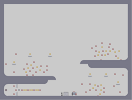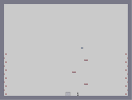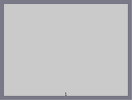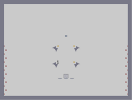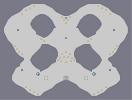No One To Hear You Scream Tile 33 - Another One With No Tiles Spooky Tile 34 - Out Of Reach 2 Spread of a Butterfly Tileset

Pages: (0)

### Gorillaz

suck!!! 4/5 cuz it looks good though

### nice

4.5/5 + .5/5 for good taste...

### yes

love the group. 5/5

### Oh yes...

The first map to be on the top rated list for N-Art lol...woo!

Sweetness.